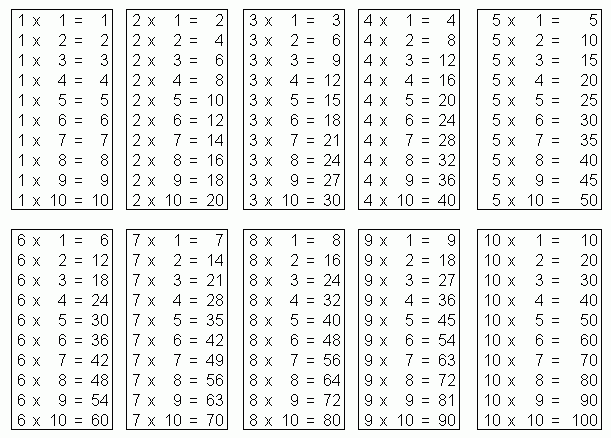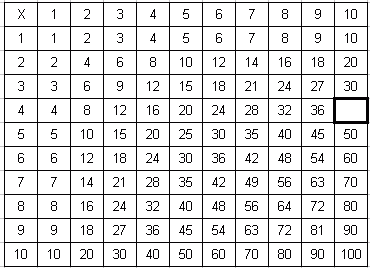Email us to get an instant 20% discount on highly effective K-12 Math & English kwizNET Programs!

#### Online Quiz (WorksheetABCD)

Questions Per Quiz = 2 4 6 8 10

### Grade 3 - Mathematics1.2 Times Tables

 Times TableMethod: Observe the rows and columns in the table carefully. Note that there is an " X " sign which denotes multiplication. The number in each row is multiplied by the corresponding number in the same column. Example: Find the missing number in the table.Look at the first number "1", in the table , multiply it with the first number in the row. This gives us 1 * 1, which is 1 and it is written under the column that reads 1. Again "1" multiplied by 2, the second element which gives 2 and written under the column that reads 2. Hence we multiply each number in the rows with the corresponding numbers in the columns. To find the missing number, it is under 2 (column - wise) , hence we multiply 5 by 2. Answer: 10 Directions: Find the missing number for the following questions, Also write the multiplication table on a sheet of paper.
 Q 1: Fill in the blank box in the table12108 Q 2: Fill in the blank box in the table403050 Question 3: This question is available to subscribers only! Question 4: This question is available to subscribers only!

#### Subscription to kwizNET Learning System offers the following benefits:

• Unrestricted access to grade appropriate lessons, quizzes, & printable worksheets
• Instant scoring of online quizzes
• Progress tracking and award certificates to keep your student motivated
• Unlimited practice with auto-generated 'WIZ MATH' quizzes